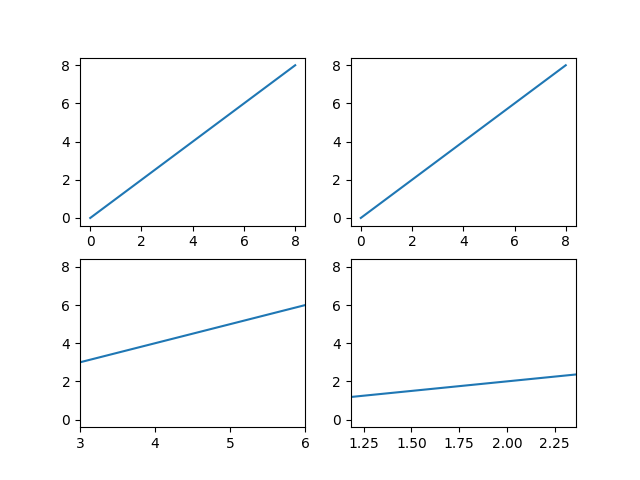# Inches and Centimeters¶

The example illustrates the ability to override default x and y units (ax1) to inches and centimeters using the xunits and yunits parameters for the plot function. Note that conversions are applied to get numbers to correct units.

This example requires basic_units.pyfrom basic_units import cm, inch
import matplotlib.pyplot as plt
import numpy as np

cms = cm * np.arange(0, 10, 2)

fig, axs = plt.subplots(2, 2)

axs[0, 0].plot(cms, cms)

axs[0, 1].plot(cms, cms, xunits=cm, yunits=inch)

axs[1, 0].plot(cms, cms, xunits=inch, yunits=cm)
axs[1, 0].set_xlim(3, 6)  # scalars are interpreted in current units

axs[1, 1].plot(cms, cms, xunits=inch, yunits=inch)
axs[1, 1].set_xlim(3*cm, 6*cm)  # cm are converted to inches

plt.show()


Keywords: matplotlib code example, codex, python plot, pyplot Gallery generated by Sphinx-Gallery